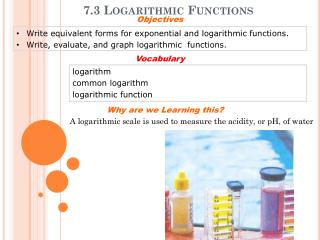DownloadDownload Presentation7.3 Logarithmic Functions

# 7.3 Logarithmic Functions

Télécharger la présentation## 7.3 Logarithmic Functions

- - - - - - - - - - - - - - - - - - - - - - - - - - - E N D - - - - - - - - - - - - - - - - - - - - - - - - - - -
##### Presentation Transcript

1. Objectives • Write equivalent forms for exponential and logarithmic functions. • Write, evaluate, and graph logarithmic functions. Vocabulary logarithm common logarithm logarithmic function Why are we Learning this? 7.3 Logarithmic Functions A logarithmic scale is used to measure the acidity, or pH, of water

2. Reading Math logba=x: “the log base b of a is x.” Notice that the log is the exponent. What Is A Log? How many times would you have to double \$1 before you had \$8? How many times would you have to double \$1 before you had \$512? 2x = 512 The inverse operation that undoes raising a base to an exponent is called finding the logarithm. Alogarithm is the exponent to which a specified base is raised to obtain a given value. A log with base 10 is called a common logarithm. If no base is written for a log, the base is assumed to be 10. For ex, log 5 = log105.

3. 1 6 1 1 2 log255 = 2 Example 1: Converting from Exponential to Logarithmic Form Write each exponential equation in logarithmic form. The base of the exponent becomes the base of the logarithm. log3243 = 5 The exponent is the logarithm. An exponent (or log) can be negative. The log (and the exponent) can be a variable.

4. 1 1 3 3 8 = 2 Example 2: Converting from Logarithmic to Exponential Form Write each logarithmic form in exponential equation. The base of the logarithm becomes the base of the power. 91 = 9 The logarithm is the exponent. 29 = 512 A logarithm can be a negative number. 1 16 Any nonzero base to the zero power is 1.

5. Evaluate by converting to an exponential statement: Log1010= Log171= A logarithm is an exponent, so the rules for exponents also apply to logs.

6. Example 3A: Evaluating Logarithms by Using Mental Math Evaluate by using mental math. log 0.01 log5 125 The log is the exponent. 10?= 0.01 Think: What power of 10 is 0.01? 10-2= 0.01 Go back to the log expression: log 0.01 = –2 1 log5 5

7. Because logarithms are the inverses of exponents, the inverse of an exponential function, such as y = 2x, is a logarithmic function, such as y = log2x. You may notice that the domain and range of each function are switched. Domain Range

8. x 0.64 0.8 1 1.25 1.5625 f–1(x) = log1.25x –2 –1 0 1 2 x –2 –1 0 1 2 1 f(x) = 1.25x 0.64 0.8 1.25 1.5625 Example 4A: Graphing Logarithmic Functions Use the x-values {–2, –1, 0, 1, 2}. Graph the function and its inverse. Describe the domain and range of the inverse function. f(x) = 1.25x Graph f(x) = 1.25x by using a table of values. To graph the inverse, f–1(x) = log1.25x,by using a table of values.